The standard gradient calculation can generate values with an unphysically large magnitude when cell quality is poor. A large gradient value in one cell can contribute suﬃciently to the source term of an equation to cause unboundedness in the solution.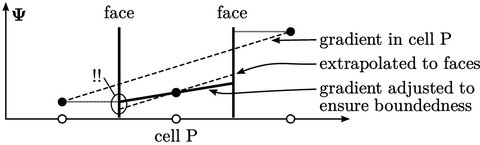The calculated gradient can be limited to avoid unphysical values. The limiting extrapolates the cell values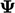using the gradient to all neighbouring face centres. If the extrapolated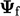at a face falls outside of the bounds of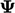at neighbouring cell centres, the gradient is adjusted to match the bounding value.

This numerical scheme has an advantage that is takes into account all surrounding cells so is fully “multi-dimensional”. It ensures boundedness locally between cells so can help eliminate instabilities in advection discretisation.

### Linear upwind with gradient limiting

Limiting can be applied to the cell gradient in the explicit contribution of the linear upwind advection scheme, described in Sec. 3.14 . The scheme with gradient limiting is analysed below for its TVD behaviour on a regular mesh in 1D.

In the following ﬁgure, the cell gradient is denoted by “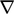” and the face gradient is denoted by “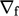”. The ratio of gradients is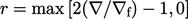from Eq. (3.12 ), which is adjusted by modifying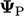. No limiting is applied for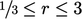, when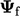can be expressed by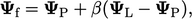with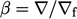. In that case,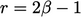, corresponding to a function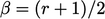.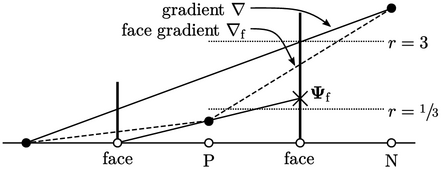Limiting is applied for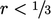, reducing the gradient linearly to zero as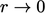. This corresponds to a function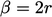for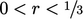. Similarly, limiting is applied when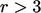. Within that range,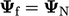so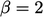.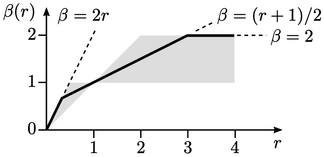On a Sweby diagram linear upwind with gradient limiting is TVD, with the line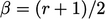being the regime with no gradient limiting.

This function uses too much downwind interpolation to be a reliable TVD scheme for advection. However, as a gradient limiter for the linear upwind scheme, it is strongly bounded, due to the multi-dimensional nature of gradient limiting — with good accuracy due to the skewness correction in linear upwind.

Notes on CFD: General Principles - 3.16 Gradient limiting